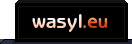# Another Visit in Hell

This game came out of a challenge posted by the regulars at the rec.games.roguelike.development newsgroup. The question was basically this - how much of a roguelike could fit inside a one-kilobyte source file?

As it turns out, quite a lot! This game features most of the staples of the genre: randomly generated dungeons, multiple enemy types, items and weapons, line of sight and magic spells (well, a magic spell). It still fits snugly under the 1024-byte limit (actually, it’s exactly at the limit). While everything had to be simplified by necessity, the game manages to be quite fun for a few plays, so I deem the whole experiment a success.

The version you can download here is actually the third attempt at cramming as much as possible into the game. The first one was called “A Journey To Hell”, and it had neither magic nor weapons. The second version introduced the weapons, while the third changed the way dungeon memory is done completely, which saved enough bytes to implement both magic and preparing your attacks to strike harder. Each time I looked at the code after a break, I found ways to make it even smaller. At this point, I’m afraid to tweak it anymore, lest it collapse into a black hole.

Binaries for Windows are available. Please look at the readme file for controls, and turn Num Lock on before playing. Or, if you don’t feel like downloading the big ZIP file, you can always copy it from the box below and run it through your trusty compiler.

``````#define F(v,h)for(v=0;v<h;v++)
#define D(i)F(y,23)F(x,T){i;}
#define G d[x][y]
#define Z(n)(n>0)-(n<0)
#define M d[x+Z(f)][y+Z(g)]
#define C(v,i)M^v||(i,M=e),
#define r rand()
int x,y,f,g,L,l=18,p=18,v,j,a,w=1,c,b,d,T=64,e=46,u=96,
K=256,k[]=L"--'*\$.@'>\$'/'\$";m(f,g){G-T?M-T||(l-=G-u)>0||(M=e):
(C(39,p+=L)C(42,l=p)C(36,v+=L*L)C(47,w=abs(L+r%4-3)+1)M>u&&(M-=
r%w+a+1)%K<=u&&(M=k[r%5+9],j++,f=g=0),M^62||N(),a=c==52);M-e?M<
T&&f*g&&(m(f,0),m(0,g)):(M=G|K,G=e);}E(t){while(x=r%T,y=r%23,G-
e);G=t;}N(){D(G=35)x=y=9;++L;F(b,21){f=r%61+2;g=r%20+1;F(c,K)G=
e,r%2?(x+=Z(f-x)):(y+=Z(g-y));}f=0;F(b,9)L<9&&E(k[b+g%2+2]);F(b
,L*2+g%3)E(99+r%(L*9/5));clear();}main(){srand(initscr());raw()
;start_color();F(b,8)init_pair(b+1,b,0);N();while(c-80){D(G%=K;
G-T||(f=x,g=y))D(F(b,8-G/u&&G^k[b]);F(c,u)d[f+(x-f)*c/u][g+(y-g